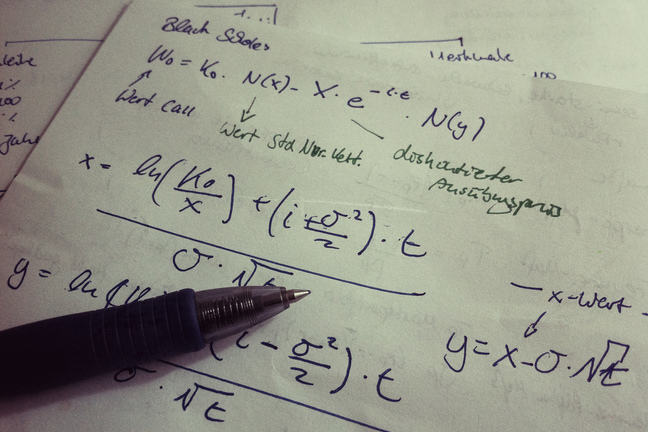3.13

## SOAS University of London# What is the Black-Scholes option pricing model?

Having looked at the features of options contracts, we can now move on to calculate the value of call options.

In the early 1970’s, Myron Scholes, Robert Merton, and Fisher Black made an important breakthrough in the pricing of complex financial instruments by developing what has become known as the Black-Scholes model. This model is used to determine the value of a call option.

The model makes some assumptions regarding the call option, that:

1. The underlying stock pays no dividends during the option’s life;
2. The options contract to be priced is a European-style call option;
3. Markets are efficient;
4. There are no commissions in the transaction;
5. Interest rates are assumed to be constant;
6. Returns of the underlying assets follow a lognormal distribution.

The model is:

$d_1$=$ln\left(\frac{S}{E}\right)+\left(r+\frac{\sigma^2}{2}\right)t\over{\sigma\sqrt{t}}$ and $d_2$=$ln\left(\frac{S}{E}\right)+\left(r-\frac{\sigma^2}{2}\right)t\over{\sigma\sqrt{t}}$$=d_1-{\sigma\sqrt{t}}$

First, let’s explain some of the variables and notation in the Black-Scholes option pricing model:

$C$ is the theoretical option value (or the price of the call option)

$S$ stands for the current stock price (assuming the underlying asset is a stock)

$E$ stands for the exercise price written in the option contract

$r$ is a risk-free interest rate (or more accurately in the Black-Scholes model it is the continuously compounded interest rate for a period of time)

$t$ is time in years until option expiration

$\sigma$ is a measure of annual volatility of the underlying stock, which is often measured by the standard deviation of stock returns (it appears in the equation as the volatility squared)

$N(d)$ refers to probability that a value less than “$d$” will occur in a standard normal distribution

$e^{rt}$ is the discount factor ($e$ = the base of natural logarithms, ie, 2.7183)

$ln$ = natural logarithm

The model is used to find the current value of a call option whose ultimate value depends on the price of the stock at the expiration date. Because the stock price keeps changing, the value of this call option will change too. Therefore, if we want to trade this option contract, then we need to use some probabilities to estimate what expected values are involved in the call option today. We need to think about the value we can expect to obtain by buying this option and what we will pay if we exercise the option.

Because the Black-Scholes option pricing model assumes that the returns on the underlying asset are normally distributed, we can make use of the standard normal distribution statistical table to find out the probability that an event will happen, and in this case the event is that we will exercise the option.

Let’s look at the Black-Scholes model more carefully:

$N(d_2)$ is the probability that the call will be exercised, so $\left(\frac{E}{e^{rt}}\right)$ $N(d_2)$ is what you expect to pay if you exercise the option, discounted to today.

And what will you get if you exercise the option? This will depend on the stock price on the expiration date (which we know will be above the exercise price if you choose to exercise the option) and on what we have assumed about the distribution of stock prices. In the equation $SN(d_1)$ is what you can expect to receive from selling the stock, if the option has been exercised, also discounted to today.

$d_1$ and $d_2$ depend on the assumptions we have made about how the stock price evolves over time, the elements in the option contract (the stock price, exercise price and time to maturity) and the other inputs – the risk-free rate and the volatility of returns (see the definitions of $d_1$ and $d_2$, respectively). The probabilities in the Black-Scholes model are functions of $d_1$ and $d_2$.

If you know $d_1$ and $d_2$, then you can find out what $N(d_1)$ and $N(d_2)$ are from the standard normal distribution table (these are the probabilities corresponding to observing values less than $d_1$ and $d_2$, respectively). With these probabilities you can then use the Black-Scholes model to obtain the option value, $C$.

As we can see, pricing an options contract using the Black-Scholes model requires many assumptions. Do you think that all these assumptions can be met in reality? Use the comments to share your thoughts.